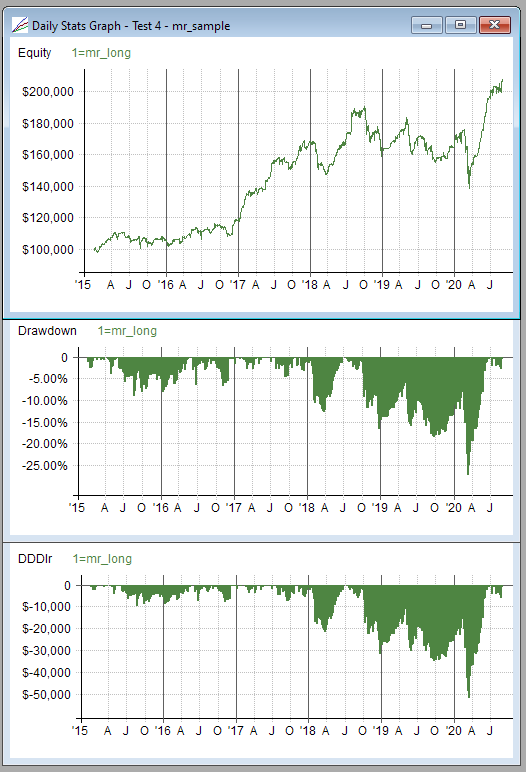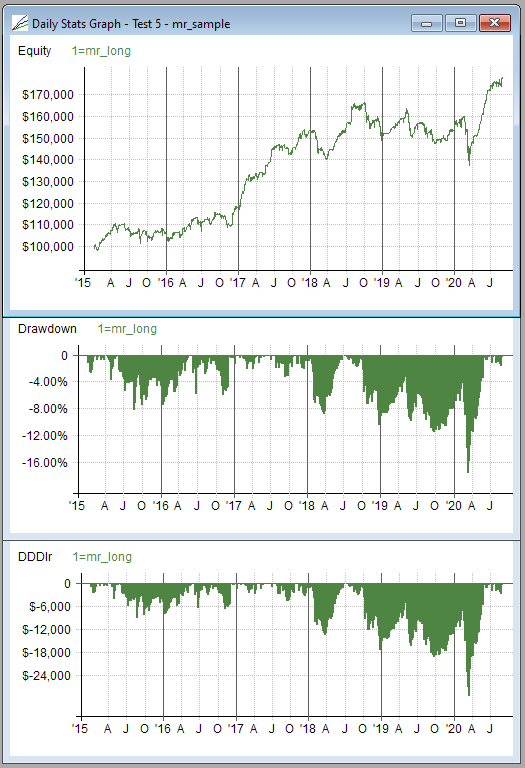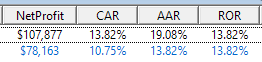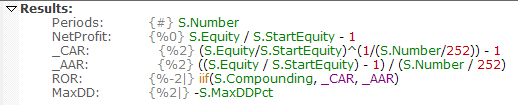Navigation: Backtest Engine Details > Compounding

RealTest automatically detects whether each strategy in a script uses compounding by looking at its Allocation and Quantity formulas.

If a strategy refers to S.Equity in its Quantity formula, or refers to S.Equity in its Allocation formula and S.Alloc in its Quantity formula, then it is considered to use compounding.

If neither of these is true, then the strategy is non-compounded.

Optionally, this heuristic can be overridden by adding a Compounded statement to a strategy.

To find out whether a strategy uses compounding, refer to S.Compounded in any formula.

If any strategy in a test uses compounding, then Combined(S.Compounded) will always be true.

This combined compounding flag is used internally to determine how some of the results statistics are reported.

When compounded is true:

rate of return (ROR) is reported as compounded annual return (CAR) of time-weighted equity

drawdown percentages are reported relative to the maximum previous equity level, i.e., ((current_equity - max_equity) / max_equity)

When compounded is false:

rate of return (ROR) is reported as average annual return (AAR)

drawdown percentages are reported relative to the starting equity level, i.e.,  ((current_equity - max_equity) / start_equity)

This automatic distinction makes it convenient to use either compounded or non-compounded equity without the typical stats distortions which are described at length in the following paragraphs.

Most backtesting software reports "compounded annual return" (CAR) and "maximum drawdown". Both of these stats, as typically reported, assume that a system was modeled to compound its equity (size each new position as a fraction of the current account balance as of the day of entry).

If a system uses a non-compounded sizing approach, such as always trading the same number of shares or the same dollar position value, then these stats designed for a compounding model make no sense. In this case, "average annual return" (AAR) is a better metric, and drawdown percentages should be calculated relative to the starting value of the account rather than its peak (or drawdowns should be shown in dollars rather than percentages).

To illustrate, consider the following example.

Here is the mr_sample.rts strategy (long side only) run from the start of 2015 through August 2020:Because this example uses compounded position sizing, though it started with a \$100K account, the \$50K drawdown in March 2020 was only a little more than 25% from the prior equity peak. As with most compounded backtests, if you compare dollar drawdown with percent drawdown, the ratio of the two gets larger as the date increases (assuming an overall profitable strategy).

Now if we run this test using non-compounded position sizing (always invest \$10K in each position), the results look like this (after temporarily changing graphs.rts to make it ignore the compounded flag and show compounded-style drawdown for this result):In this case, the \$25K+ drawdown is reported as only a bit more than 16%.

This is the correct percentage based on the equity peak, but it doesn't make sense to report it this way when the model was still using only \$100K of capital at that point in the test.

Changing the drawdown formula back to the correct version, the drawdown percentage graph now looks like this:In other words, very similar to the same graph from the compounded test.

Looking at the summary results statistics, the default column definitions only include ROR, but here I've added CAR and AAR to illustrate this point:For the first (compounded) test, the CAR figure makes sense but the AAR value does not (which shows why mutual funds love to report average annual return...)

Conversely, for the second (non-compounded) test, the CAR figure understates the results.

By default, RealTest only includes the ROR column, which automatically displays the appropriate statistic for the compounding mode used in each backtest.

How this is done can be seen by looking at the Results.RTS script:(Items that start with underscores are not displayed as columns but can be used to store intermediate values. To produce the output shown above I just temporarily removed the underscores.)

Calculating overall MaxDD percentage is a bit trickier, because it relies (when compounded) on knowing what the peak equity value was prior to the drawdown. This is handled internally, to always return the correct value in the S.MaxDDPct stat.